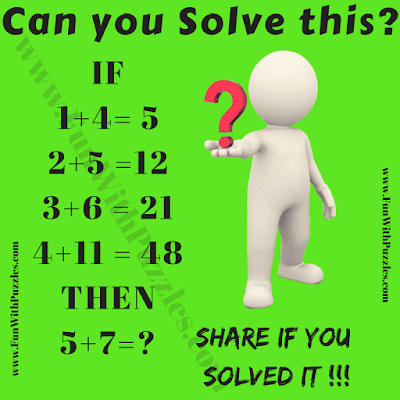Time to solve this Fun Mathematical Logic Riddle. This is Maths Logic Riddle in which there are some Maths equations. Your challenge is to find the logic in these Maths equations and then find the missing number which will replace the question mark. So are you ready to Crack this Fun Mathematical Logic Riddle?Can you solve this Fun Maths Logic Riddle?

Answer of this "Fun Mathematical Logic Riddle", can be viewed by clicking on button. Please do give your best try before looking at the answer.

Unknown said...

sairam said...

sairam said...